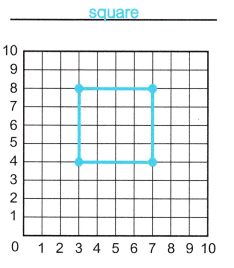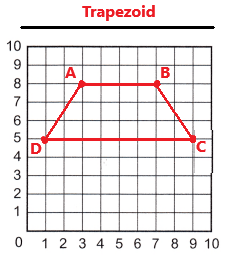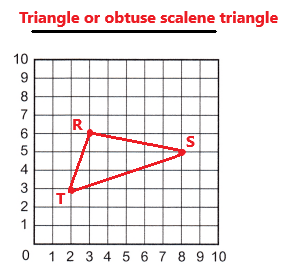# McGraw Hill Math Grade 5 Chapter 11 Lesson 4 Answer Key Plotting Points to Form Lines and Shapes

All the solutions provided in McGraw Hill Math Grade 5 Answer Key PDF Chapter 11 Lesson 4 Plotting Points to Form Lines and Shapes are as per the latest syllabus guidelines.

## McGraw-Hill Math Grade 5 Answer Key Chapter 11 Lesson 4 Plotting Points to Form Lines and Shapes

Plot and Draw

Plot each set of points. Draw lines between the points. Identify the shape.

Question 1.
A (3, 8)
B (7, 8)
C (7, 4)
D (3, 4)
Identify the shape.
_____________Explanation:
After plotting and joining the given set of points we can see that a quadrilateral having four equal sides. So, this is a Square.

Question 2.
A (3, 8)
B (7, 8)
C (9, 5)
D (1, 5)
Identify the shape.
_______________Explanation:
After plotting and joining the given set of points we can see that a quadrilateral with a pair of parallel sides is formed without any right angles. So, this is a trapezoid.

Question 3.
R (3, 6)
S (8, 5)
T (2, 3)
Identify the shape.
______________